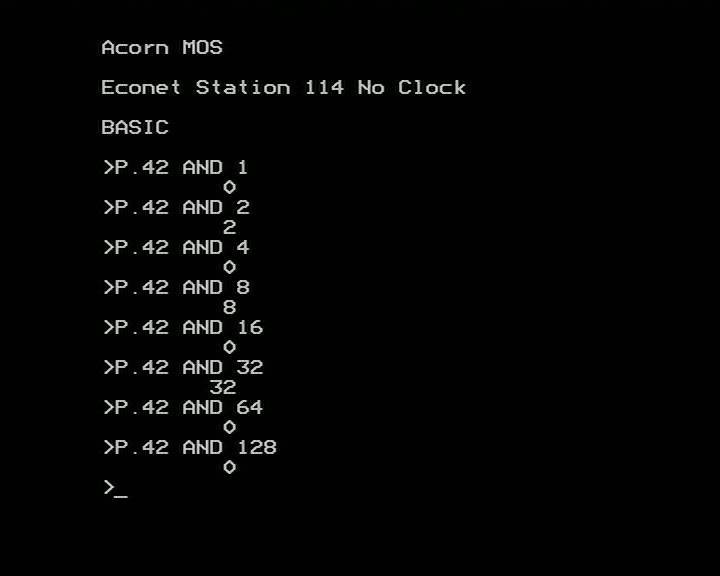Working with BinaryVery often it is helpful to be able to extract the contents of each of the bits in a byte - by doing so, we can use a single byte of storage to hold 8 separate flags. To do this, we take the number in question and AND it with each power of two starting from 1. If the result is zero it means the bit is clear. If the result is the number we ANDed with, it means the bit it set.

In the example above, 42 is made up of 3 set bits and 5 clear bits. The set bits correspond to bits 1 (holding a decimal value of 2), 3 (holding a decimal value of 8) and 5 (holding a decimal value of 32).